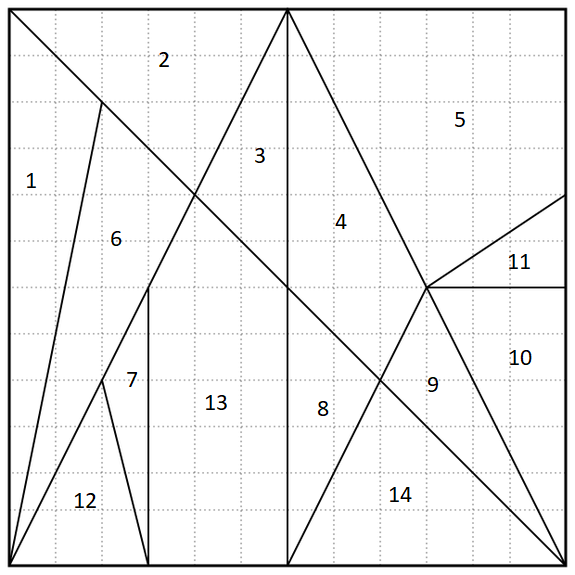The famous four-color theorem states, essentially, that you can color in the regions of any map using at most four colors in such a way that no neighboring regions share a color. A computer-based proof of the theorem was offered in 1976.

Some 2,200 years earlier, the legendary Greek mathematician Archimedes described something called an Ostomachion. It’s a group of pieces, similar to tangrams, that divides a 12-by-12 square into 14 regions. The object is to rearrange the pieces into interesting shapes, such as a Tyrannosaurus rex. It’s often called the oldest known mathematical puzzle.

Your challenge today: Color in the regions of the Ostomachion square with four colors such that each color shades an equal area. (That is, each color needs to shade 36 square units.)

(fivethirtyeight)## Solution

See the previous post to learn more about the operation of Google’s Constraint Solver that is used here as well. In a flash we learn that there are 9 solutions with all four colors used and two with only three of the four used. That’s counting solutions as equivalent if colors are merely swapped.

# We use Google's Constraint Programming solver to solve the puzzle in a flash.
# Find it at https://developers.google.com/optimization/cp/
from ortools.constraint_solver import pywrapcp
from itertools import permutations

Cells = tuple(range(1,15))
Areas = (0,12,12,6,12,24,12,3,6,6,9,3,6,21,12)
Walls = ((1,2),(1,6),(2,3),(2,6),(3,4),(3,13),(4,5),(4,8),(4,9),(5,11),(6,7),(6,12),(6,13),(7,12),(7,13),(8,13),(8,14),(9,10),(9,14),(10,11))
NColors = 4
Colors = tuple(range(NColors))

# Create the solver.
solver = pywrapcp.Solver("")

# Create the variables.
Vars = []
Color = {}
for Cell in Cells:
Color[Cell] = solver.IntVar(0,len(Colors)-1,"Color_"+str(Cell))
Vars.append(Color[Cell])

# Constraints
for Col in Colors:
c = (sum([Areas[Cell]*(Color[Cell]==Col) for Cell in Cells]) == 36)
solver.Add(c)
for Wall in Walls:
solver.Add(solver.AllDifferent((Color[Wall],Color[Wall])))

# Create the "decision builder"
db = solver.Phase(Vars, solver.CHOOSE_FIRST_UNBOUND, solver.ASSIGN_MIN_VALUE)

# Call the solver and display the unique solutions.
Solutions = []
def NewSolution():
for Solution in Solutions:
for Permute in permutations(Colors):
Same = True
for Cell in Cells:
if not Color[Cell].Value() == Permute[Solution[Cell-1]]:
Same = False
if Same:
return False
return True

if solver.Solve(db):
Count = 0
while solver.NextSolution():
if NewSolution():
Count += 1
Solution = [Color[Cell].Value() for Cell in Cells]
Solutions.append(Solution)
print("Solution",Count,Solution)
else:
print("No solution found.")


The output:

Solution 1 [0, 1, 0, 1, 2, 2, 0, 0, 0, 3, 0, 3, 3, 1]
Solution 2 [0, 1, 0, 1, 2, 2, 0, 0, 3, 0, 3, 3, 3, 1]
Solution 3 [0, 1, 0, 1, 2, 2, 1, 0, 0, 1, 3, 0, 3, 3]
Solution 4 [0, 1, 0, 2, 1, 2, 0, 0, 0, 3, 0, 3, 3, 2]
Solution 5 [0, 1, 0, 2, 1, 2, 0, 0, 3, 0, 3, 3, 3, 2]
Solution 6 [0, 1, 0, 2, 3, 2, 0, 0, 3, 0, 1, 3, 1, 2]
Solution 7 [0, 1, 0, 2, 3, 2, 3, 0, 0, 3, 1, 0, 1, 2]
Solution 8 [0, 1, 0, 2, 3, 3, 1, 0, 0, 1, 2, 0, 2, 1]
Solution 9 [0, 1, 0, 2, 3, 3, 2, 0, 0, 2, 1, 0, 1, 2]
[Finished in 0.3s]